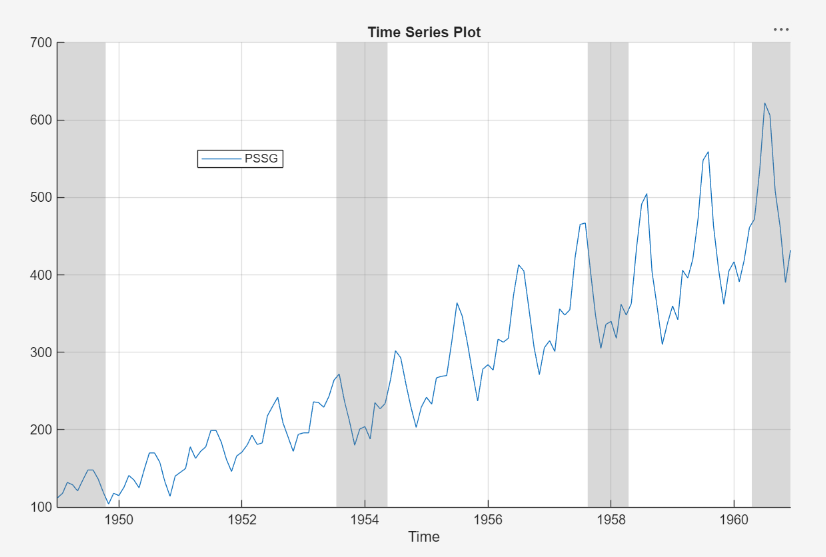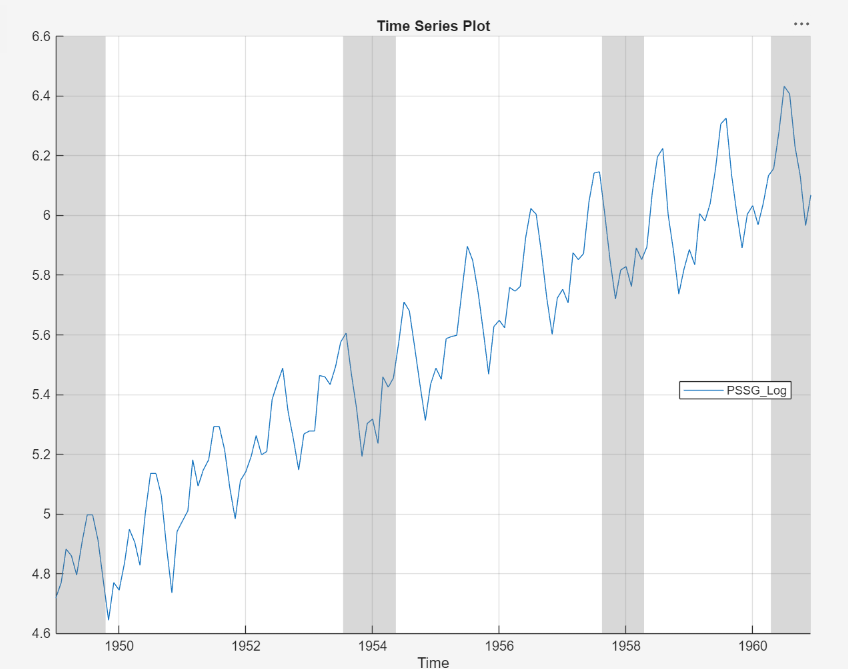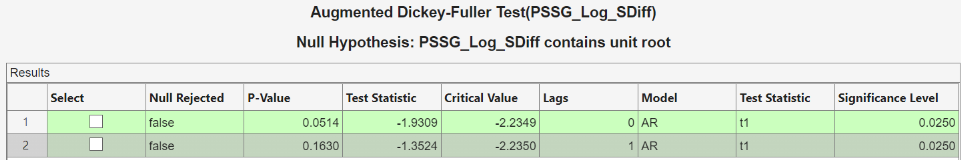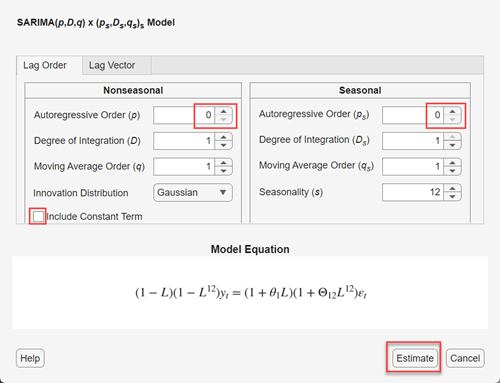## Estimate Multiplicative ARIMA Model Using Econometric Modeler App

This example uses the Box-Jenkins method  to determine a multiplicative seasonal ARIMA (SARIMA) model for a univariate series by using the Econometric Modeler app. Specifically, the example shows this procedure:

1. Visually determine whether the series has an exponential trend, and then remove the trend if one is present.

The remaining steps determine the structure of the conditional mean model to fit to the series without the exponential trend.

2. Visually determine whether the transformed series has seasonal integration, and then remove the seasonal integration, if it is present, by taking the seasonal difference. Record the degrees of seasonal integration removed.

3. Determine whether the transformed series has a unit root by conducting a statistical test, and then apply the first difference to the series to stabilize it, if a unit root is present. Record whether a unit root exists.

4. Determine the nonseasonal AR and MA degrees of the conditional mean model for the stationary series by inspecting correlograms of the stabilized series. Record the polynomial degrees.

5. Use the results of steps 2 through 4 to create and estimate a conditional mean model for the nonstationary series with the exponential trend removed.

The data set `Data_Airline.mat` contains monthly counts of airline passengers.

### Import Data into Econometric Modeler

At the command line, load the `Data_Airline.mat` data set.

`load Data_Airline`

At the command line, open the Econometric Modeler app.

`econometricModeler`

Alternatively, open the app from the apps gallery (see Econometric Modeler).

Import `DataTimeTable` into the app:

1. On the Econometric Modeler tab, in the Import section, click the button.

2. In the Import Data dialog box, in the Import? column, select the check box for the `DataTimeTable` variable.

3. Click .

The variable `PSSG` appears in the Time Series pane, its value appears in the Preview pane, and its time series plot appears in the Time Series Plot(PSSG) figure window.The series exhibits a seasonal trend, serial correlation, and possible exponential growth. For an interactive analysis of serial correlation, see Detect Serial Correlation Using Econometric Modeler App.

### Stabilize Series

To properly determine the lag operator polynomial orders for the conditional mean model, correlograms require a stationary series.

Address the exponential trend by applying the log transform to `PSSG`.

1. In the Time Series pane, select `PSSG`.

2. On the Econometric Modeler tab, in the Transforms section, click .

The transformed variable `PSSGLog` appears in the Time Series pane, its value appears in the Preview pane, and its time series plot appears in the Time Series Plot(PSSGLog) figure window.The exponential growth appears to be removed from the series.

The rest of the example determines the parameters of conditional mean model for the series `PSSGLog` by several visual and statistical diagnoses and transformations.

Address the seasonal trend by applying the 12th order seasonal difference. With `PSSGLog` selected in the Time Series pane, on the Econometric Modeler tab, in the Transforms section, set Seasonal to `12`. Then, click .

The transformed variable `PSSGLogSeasonalDiff` appears in the Time Series pane, and its time series plot appears in the Time Series Plot(PSSGLogSeasonalDiff) figure window.The transformed series appears to have a unit root.

Test the null hypothesis that `PSSGLogSeasonalDiff` has a unit root by using the Augmented Dickey-Fuller test. Specify that the alternative is an AR(0) model, then test again specifying an AR(1) model. Adjust the significance level to 0.025 to maintain a total significance level of 0.05.

1. With `PSSGLogSeasonalDiff` selected in the Time Series pane, on the Econometric Modeler tab, in the Tests section, click > Augmented Dickey-Fuller Test.

2. On the ADF tab, in the Parameters section, set Significance Level to `0.025`.

3. In the Tests section, click .

4. In the Parameters section, set Number of Lags to `1`.

5. In the Tests section, click .

The test results appear in the Results table of the ADF(PSSGLogSeasonalDiff) document.Both tests fail to reject the null hypothesis that the series is a unit root process.

Address the unit root by applying the first difference to `PSSGLogSeasonalDiff`. With `PSSGLogSeasonalDiff` selected in the Time Series pane, click the Econometric Modeler tab. Then, in the Transforms section, click .

The transformed variable `PSSGLogSeasonalDiffDiff` appears in the Time Series pane, and its time series plot appears in the Time Series Plot(PSSGLogSeasonalDiffDiff) figure window.

In the Time Series pane, rename the `PSSGLogSeasonalDiffDiff` variable by clicking it twice to select its name and entering `PSSGStable`.

The app updates the names of all documents associated with the transformed series.### Identify Model for Series

Determine the lag structure for a conditional mean model of the series by plotting the sample autocorrelation function (ACF) and partial autocorrelation function (PACF) of the stabilized series.

1. With `PSSGStable` selected in the Time Series pane, click the Plots tab, then click .

2. Show the first 50 lags of the ACF. On the ACF tab, set Number of Lags to `50`.

3. Click the Plots tab, then click .

4. Show the first 50 lags of the PACF. On the PACF tab, set Number of Lags to `50`.

5. Drag the ACF(PSSGStable) figure window above the PACF(PSSGStable) figure window.According to , the autocorrelations in the ACF and PACF, the results in previous steps, and the balance of model parsimony with complexity, suggest that the following SARIMA(0,1,1)×(0,1,1)12 model is appropriate for the nonstationary series with the exponential trend removed `PSSGLog`.

`$\left(1-L\right)\left(1-{L}^{12}\right){y}_{t}=\left(1+{\theta }_{1}L\right)\left(1+{\Theta }_{12}{L}^{12}\right){\epsilon }_{t}.$`

Close all figure windows.

### Specify and Estimate SARIMA Model

Specify the SARIMA(0,1,1)×(0,1,1)12 model.

1. In the Time Series pane, select the `PSSGLog` time series.

2. On the Econometric Modeler tab, in the Models section, click the arrow > SARIMA.

3. In the SARIMA Model Parameters dialog box, on the Lag Order tab:

• Nonseasonal section

1. Set Degrees of Integration to `1`.

2. Set Moving Average Order to `1`.

3. Clear the Include Constant Term check box.

• Seasonal section

1. Set Period to `12` to indicate monthly data.

2. Set Moving Average Order to `1`.

3. Select the Include Seasonal Difference check box.4. Click .

The model variable `SARIMA_PSSGLog` appears in the Models pane, its value appears in the Preview pane, and its estimation summary appears in the Model Summary(SARIMA_PSSGLog) document.The results include:

• Model Fit — A time series plot of `PSSGLog` and the fitted values from `SARIMA_PSSGLog`.

• Residual Plot — A time series plot of the residuals of `SARIMA_PSSGLog`.

• Parameters — A table of estimated parameters of `SARIMA_PSSGLog`. Because the constant term was held fixed to 0 during estimation, its value and standard error are 0.

• Goodness of Fit — The AIC and BIC fit statistics of `SARIMA_PSSGLog`.

 Box, George E. P., Gwilym M. Jenkins, and Gregory C. Reinsel. Time Series Analysis: Forecasting and Control. 3rd ed. Englewood Cliffs, NJ: Prentice Hall, 1994.